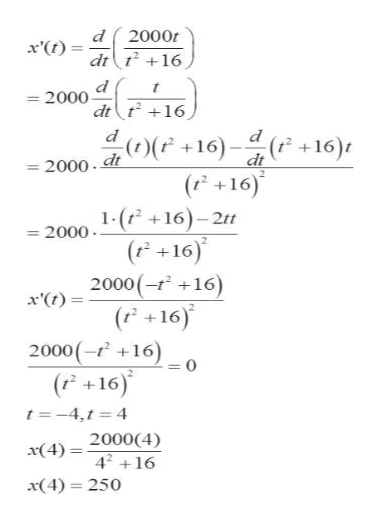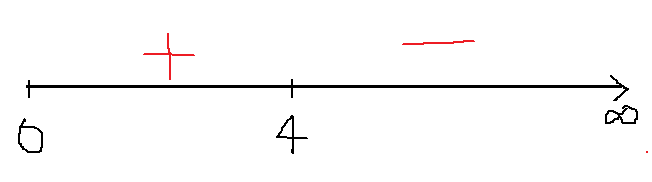# The number of miligrams x of a medication in the bloodstream t hours after a dose is taken can be modeled byx(t)= 2000t/t^2+16(a) For what t-values is x increasing?(b) Find the t-value at which s is maximum.(c) Fine the maximum value of x.

Question
3 views

The number of miligrams x of a medication in the bloodstream t hours after a dose is taken can be modeled by

x(t)= 2000t/t^2+16

(a) For what t-values is x increasing?

(b) Find the t-value at which s is maximum.

(c) Fine the maximum value of x.

check_circle

Step 1

we are given a function

Step 2

Now, we will find derivative and then we can set derivative to 0

and then we can solve for t

and then we find point  and time can never be negative

so, we will only consider t=4help_outlineImage Transcriptionclose2000 dr t16 x'(t) 2000 dtt16 d 0(F+16) ( +16)t dt -2000. dt (P+16) 2000. 1-(16)-2ft (+16) 2000 (-r16) x'(t (P+16) 2000(-16) (r+16) t-4,t 4 x(4) =2000(4) 42+16 x(4) 250 fullscreen
Step 3

Now, we can draw a number line and locate t=4

and then we can find sign of derivative on each intervals...

### Want to see the full answer?

See Solution

#### Want to see this answer and more?

Solutions are written by subject experts who are available 24/7. Questions are typically answered within 1 hour.*

See Solution
*Response times may vary by subject and question.
Tagged in

### Derivative